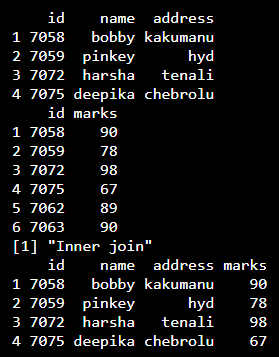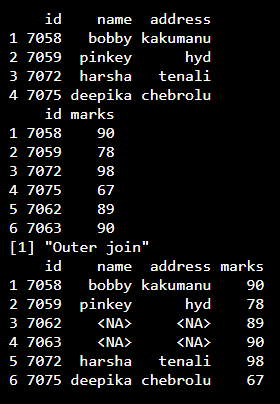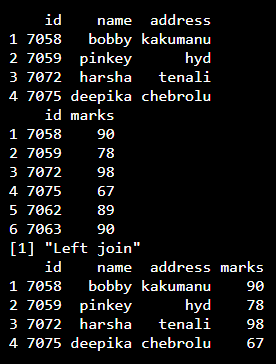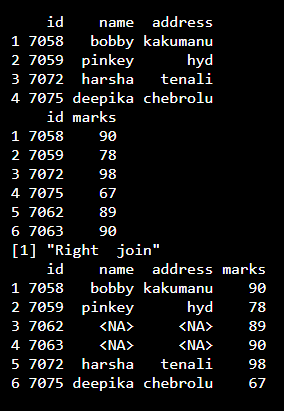# How to merge dataframes in R ?

• Last Updated : 09 May, 2021

In this article, we will discuss how to perform inner, outer, left, or right joins in a given dataframe in R Programming Language.

### Functions Used

merge() function is used to merge or join two tables. With appropriate values provided to specific parameters, we can create the desired join.

Syntax: merge(df1, df2, by.df1, by.df2, all.df1, all.df2, sort = TRUE)

Parameters:

df1: one dataframe
df2: another dataframe
by.df1, by.df2: The names of the columns that are common to both df1 and df2.
all, all.df1, all.df2: Logical values that actually specify the type of merging happens.

### Inner join

An inner join also known as natural join, merges the two dataframes in one that contains the common elements of both. For this merge() function is simply given the values of two dataframes in consideration and on the basis of a common column a dataframe is generated.Syntax:

merge(x = dataframe 1, y = data frame 2)

Example

## R

 `# create data frame 1 with id ,``# name and address ``df1=``data.frame``(id=``c``(7058,7059,7072,7075), ``               ``name=``c``(``"bobby"``,``"pinkey"``,``"harsha"``,``"deepika"``),``               ``address=``c``(``"kakumanu"``,``"hyd"``,``"tenali"``,``"chebrolu"``))`` ` `# create data frame 2 with id , ``# marks  ``df2=``data.frame``(id=``c``(7058,7059,7072,7075,7062,7063), ``               ``marks=``c``(90,78,98,67,89,90))`` ` `# display dataframe1``print``(df1)`` ` `# display dataframe2``print``(df2)`` ` `print``(``"Inner join"``)`` ` `# inner join ``print``(``merge``(x = df1, y = df2))`

Output:### Outer Join

Outer Join merges all the columns of both data frames into one for all elements. For this, the dataframes in consideration along with all parameter assigned value TRUE has to be passed to merge() function.Syntax:

merge(x = data frame 1, y = data frame 2, all = TRUE)

Example:

## R

 `# create data frame 1 with id , name and address ``df1=``data.frame``(id=``c``(7058,7059,7072,7075), ``               ``name=``c``(``"bobby"``,``"pinkey"``,``"harsha"``,``"deepika"``),``               ``address=``c``(``"kakumanu"``,``"hyd"``,``"tenali"``,``"chebrolu"``))`` ` `# create data frame 2 with id , marks  ``df2=``data.frame``(id=``c``(7058,7059,7072,7075,7062,7063), ``               ``marks=``c``(90,78,98,67,89,90))`` ` `# display dataframe1``print``(df1)`` ` `# display dataframe2``print``(df2)`` ` `print``(``"Inner join"``)`` ` `# outer join ``print``(``merge``(x = df1, y = df2,all=``TRUE``))`

Output:Note: It returns NA of unmatched columns

### Left Join

It gives the data which are matching all the rows in the first data frame with the corresponding values on the second data frame. For this along with the dataframes in consideration, all parameter has to be passed TRUE after giving reference of the left table.Syntax:

merge(x = data frame 1, y = data frame 2, all.x = TRUE)

Example:

## R

 `# create data frame 1 with id , name and address ``df1=``data.frame``(id=``c``(7058,7059,7072,7075), ``               ``name=``c``(``"bobby"``,``"pinkey"``,``"harsha"``,``"deepika"``),``               ``address=``c``(``"kakumanu"``,``"hyd"``,``"tenali"``,``"chebrolu"``))`` ` `# create data frame 2 with id , marks  ``df2=``data.frame``(id=``c``(7058,7059,7072,7075,7062,7063), ``               ``marks=``c``(90,78,98,67,89,90))`` ` `# display dataframe1``print``(df1)`` ` `# display dataframe2``print``(df2)`` ` `print``(``"Left join"``)``# Left join ``print``(``merge``(x = df1, y = df2,all.x=``TRUE``))`

Output:### Right Join

It gives the data which are matching all the rows in the second data frame with the corresponding values on the first data frame. For this merge() function should be provided with dataframes along with all parameters assigned TRUE. all parameters should have a reference to the right dataframe.Syntax:

merge(x = data frame 1, y = data frame 2, all.y = TRUE)

Example:

## R

 `# create data frame 1 with id , name and address ``df1=``data.frame``(id=``c``(7058,7059,7072,7075), ``               ``name=``c``(``"bobby"``,``"pinkey"``,``"harsha"``,``"deepika"``),``               ``address=``c``(``"kakumanu"``,``"hyd"``,``"tenali"``,``"chebrolu"``))`` ` `# create data frame 2 with id , marks  ``df2=``data.frame``(id=``c``(7058,7059,7072,7075,7062,7063), ``               ``marks=``c``(90,78,98,67,89,90))`` ` `# display dataframe1``print``(df1)`` ` `# display dataframe2``print``(df2)`` ` `print``(``"Right  join"``)``# Right  join ``print``(``merge``(x = df1, y = df2,all.y=``TRUE``))`

Output:My Personal Notes arrow_drop_up